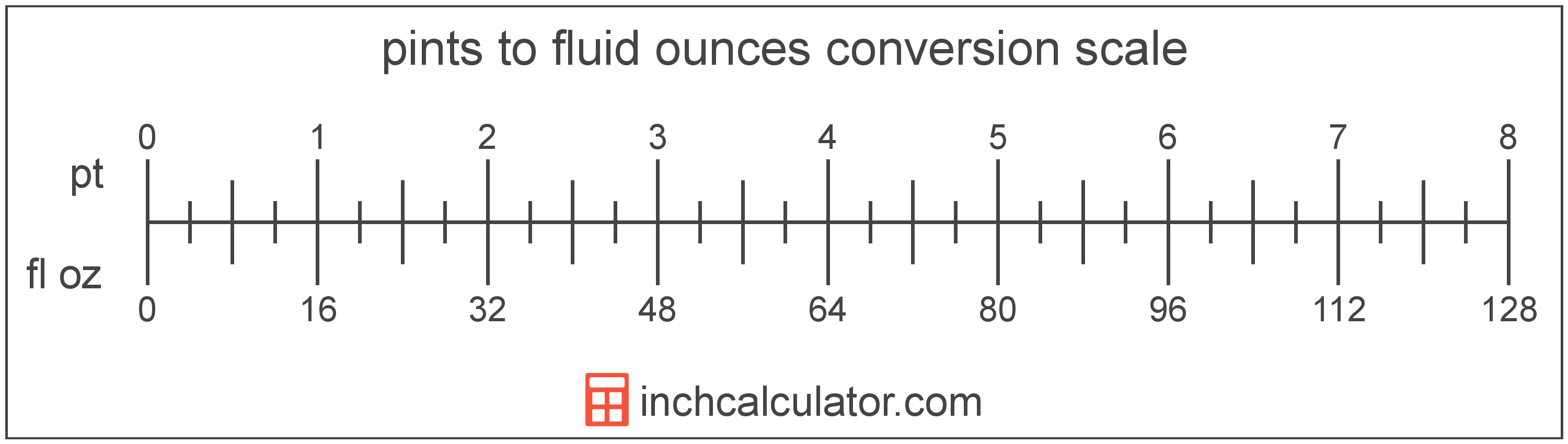# Pints to Fluid Ounces Converter

Enter the volume in pints below to get the value converted to fluid ounces.

Results in Fluid Ounces:1 pt = 16 fl oz

Do you want to convert fluid ounces to pints?

## How to Convert Pints to Fluid Ounces

To convert a measurement in pints to a measurement in fluid ounces, multiply the volume by the following conversion ratio: 16 fluid ounces/pint.

Since one pint is equal to 16 fluid ounces, you can use this simple formula to convert:

fluid ounces = pints × 16

The volume in fluid ounces is equal to the volume in pints multiplied by 16.

For example, here's how to convert 5 pints to fluid ounces using the formula above.
fluid ounces = (5 pt × 16) = 80 fl oz### How Many Fluid Ounces Are in a Pint?

There are 16 fluid ounces in a pint, which is why we use this value in the formula above.

1 pt = 16 fl oz

## What is a Pint?

The US liquid pint is a unit of fluid volume equal to one-eighth of a gallon, one-half of a quart, or two cups. The liquid pint should not be confused with the dry pint (US) or the imperial pint, which are different units.

The pint is a US customary unit of volume. Pints can be abbreviated as pt; for example, 1 pint can be written as 1 pt.

## What is a Fluid Ounce?

The US fluid ounce is a unit of volume equal to 1/16 of a pint or 1/8 of a cup. The fluid ounce is sometimes referred to as just an "ounce" but should not be confused with the unit of weight. One fluid ounce is equal to approximately 29.5735 milliliters, but in nutrition labeling, one fluid ounce is rounded to exactly 30 milliliters.

The fluid ounce is a US customary unit of volume. Fluid ounces can be abbreviated as fl oz, and are also sometimes abbreviated as oz fl. For example, 1 fluid ounce can be written as 1 fl oz or 1 oz fl.

## Pint to Fluid Ounce Conversion Table

Table showing various pint measurements converted to fluid ounces.
Pints Fluid Ounces
1 pt 16 fl oz
2 pt 32 fl oz
3 pt 48 fl oz
4 pt 64 fl oz
5 pt 80 fl oz
6 pt 96 fl oz
7 pt 112 fl oz
8 pt 128 fl oz
9 pt 144 fl oz
10 pt 160 fl oz
11 pt 176 fl oz
12 pt 192 fl oz
13 pt 208 fl oz
14 pt 224 fl oz
15 pt 240 fl oz
16 pt 256 fl oz
17 pt 272 fl oz
18 pt 288 fl oz
19 pt 304 fl oz
20 pt 320 fl oz
21 pt 336 fl oz
22 pt 352 fl oz
23 pt 368 fl oz
24 pt 384 fl oz
25 pt 400 fl oz
26 pt 416 fl oz
27 pt 432 fl oz
28 pt 448 fl oz
29 pt 464 fl oz
30 pt 480 fl oz
31 pt 496 fl oz
32 pt 512 fl oz
33 pt 528 fl oz
34 pt 544 fl oz
35 pt 560 fl oz
36 pt 576 fl oz
37 pt 592 fl oz
38 pt 608 fl oz
39 pt 624 fl oz
40 pt 640 fl oz

## References

1. U.S. Food & Drug Administration, Guidance for Industry: Guidelines for Determining Metric Equivalents of Household Measures, https://www.fda.gov/regulatory-information/search-fda-guidance-documents/guidance-industry-guidelines-determining-metric-equivalents-household-measures